# Exceptional analytic set

An analytic setin a complex spacefor which there exists an analytic mappingsuch that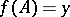is a point in the complex space, whileis an analytic isomorphism. The modificationis called a contraction ofto.
The problem of characterizing exceptional sets arose in algebraic geometry in relation to the study of birational transformations (cf. Birational transformation and also Exceptional subvariety). Very general criteria for exceptional sets have been found in analytic geometry. More precisely, letbe a connected compact analytic set of positive dimension in a complex space. The setis exceptional if and only if there is a relatively-compact pseudo-convex neighbourhood of it inin which it is a maximal compact analytic subset.
Let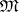be a coherent sheaf of ideals whose zero set coincides withand letbe the restriction toof the linear space overdual to(cf. Vector bundle, analytic). Forto be exceptional it is sufficient thatbe weakly negative (cf. Positive vector bundle). Ifis a manifold andis a submanifold of it, thenis the normal bundle over. Sometimes, the bundlebeing weakly negative is also necessary (e.g. ifis a submanifold of codimension 1, isomorphic to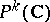, or ifis a two-dimensional manifold). In particular, a curveon a complex surfaceis exceptional if and only if the intersection matrixof its irreducible components is negative definite (cf. , ). The structure of a neighbourhood of an exceptional analytic set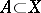is completely determined by the ringed space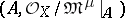for sufficiently large. Exceptional analytic sets have the following transitiveness condition: If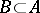is a compact analytic space inand is exceptional in, whileis exceptional in, thenis exceptional in. There are relative generalizations of the concept of an exceptional analytic set. These consider, roughly speaking, the simultaneous contraction of a family of analytic sets in an analytic family of complex spaces. An analogue of Grauert's criterion mentioned above is valid in this case (cf. ).
Another natural generalization of the concept of an exceptional analytic set is as follows. Letbe a subspace inand let a proper surjective holomorphic mapping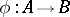be given. A contraction ofalongis a proper surjective holomorphic mapping, wherecontainsas a subspace, such that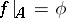andinduces an isomorphism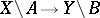. Ifis a manifold of dimension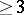,is a compact submanifold of codimension one in it, andis a fibration with fibre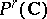,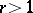, then a necessary and sufficient condition forto be contractible alongonto a manifoldis: The normal bundleover(which in this case coincides with the bundle corresponding to the divisor) must induce a bundle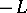on each fibre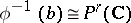, whereis determined by a hyperplane in. The corresponding contraction is the inverse to the monoidal transformation with centre at(cf. ). On the other hand, for each modification, whereis a manifold,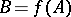is a submanifold of it,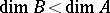, and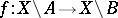is an isomorphism, the mapping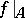is a fibration with fibre. Criteria for contractibility along, as well as in more general situations, are known (cf. ). Ifis exceptional inand is a holomorphic retract of it (e.g.is the zero section of a weakly-negative vector bundle), thenhas a contraction along any. If, moreover, the dimensions of the fibres of the retract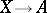are equal to at least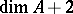, one can completely recover the initial space from the dataobtained after contraction .